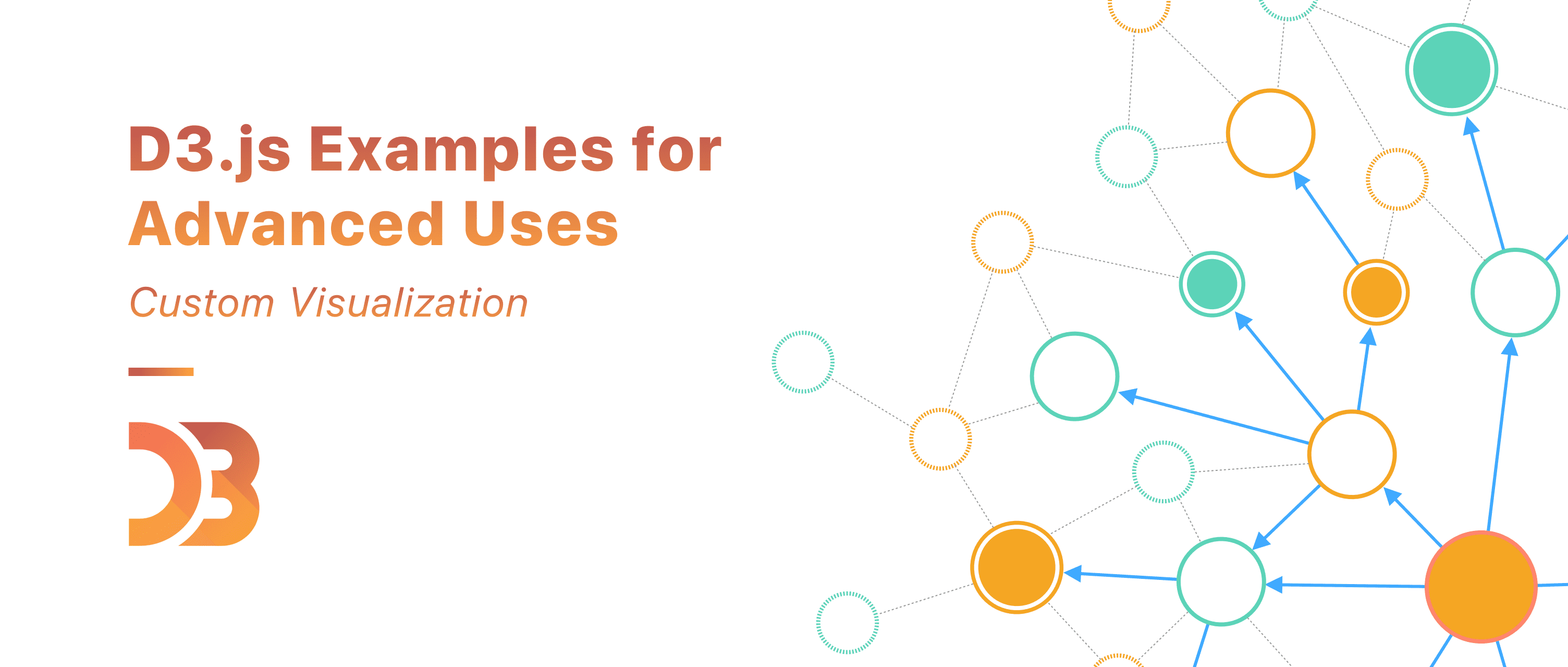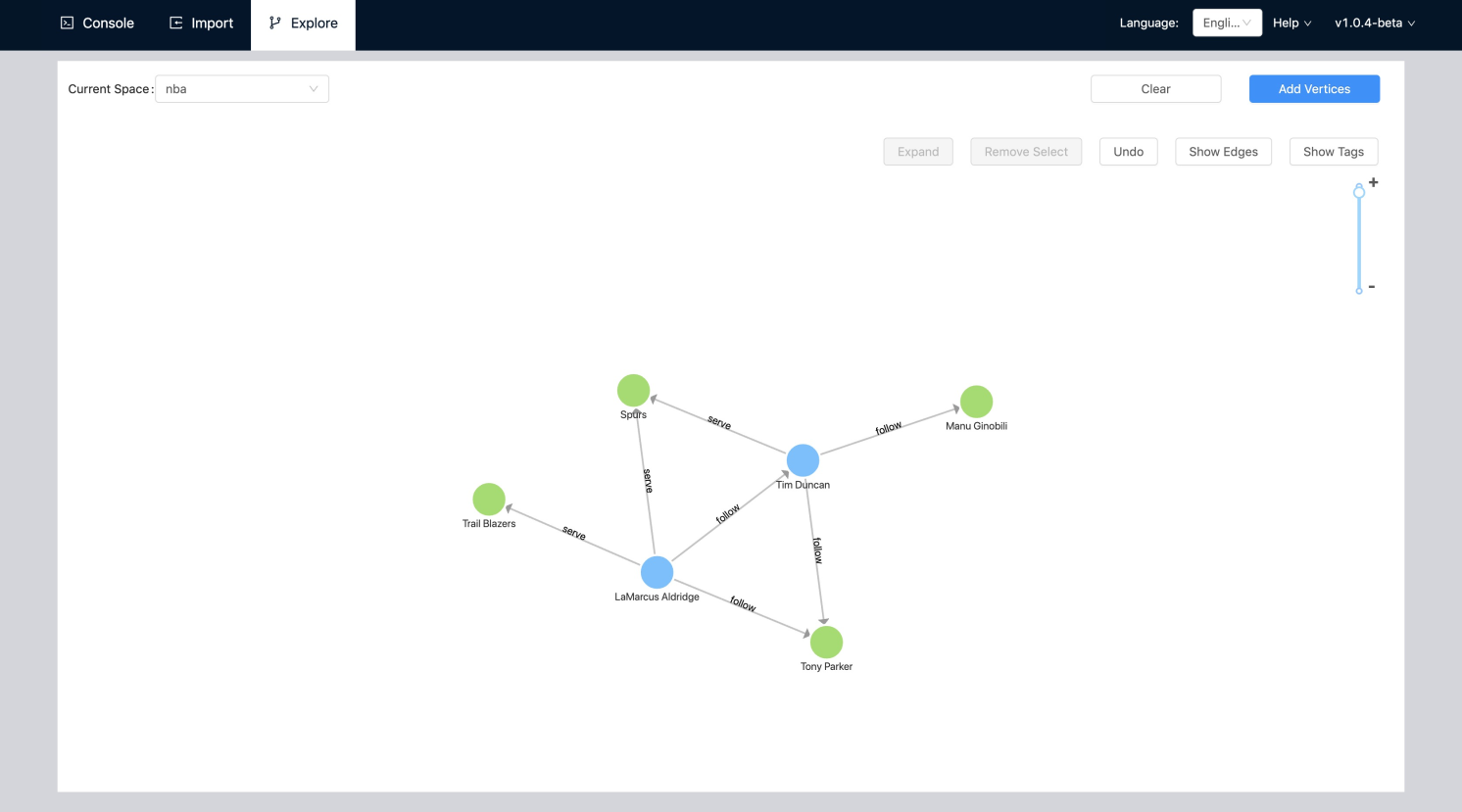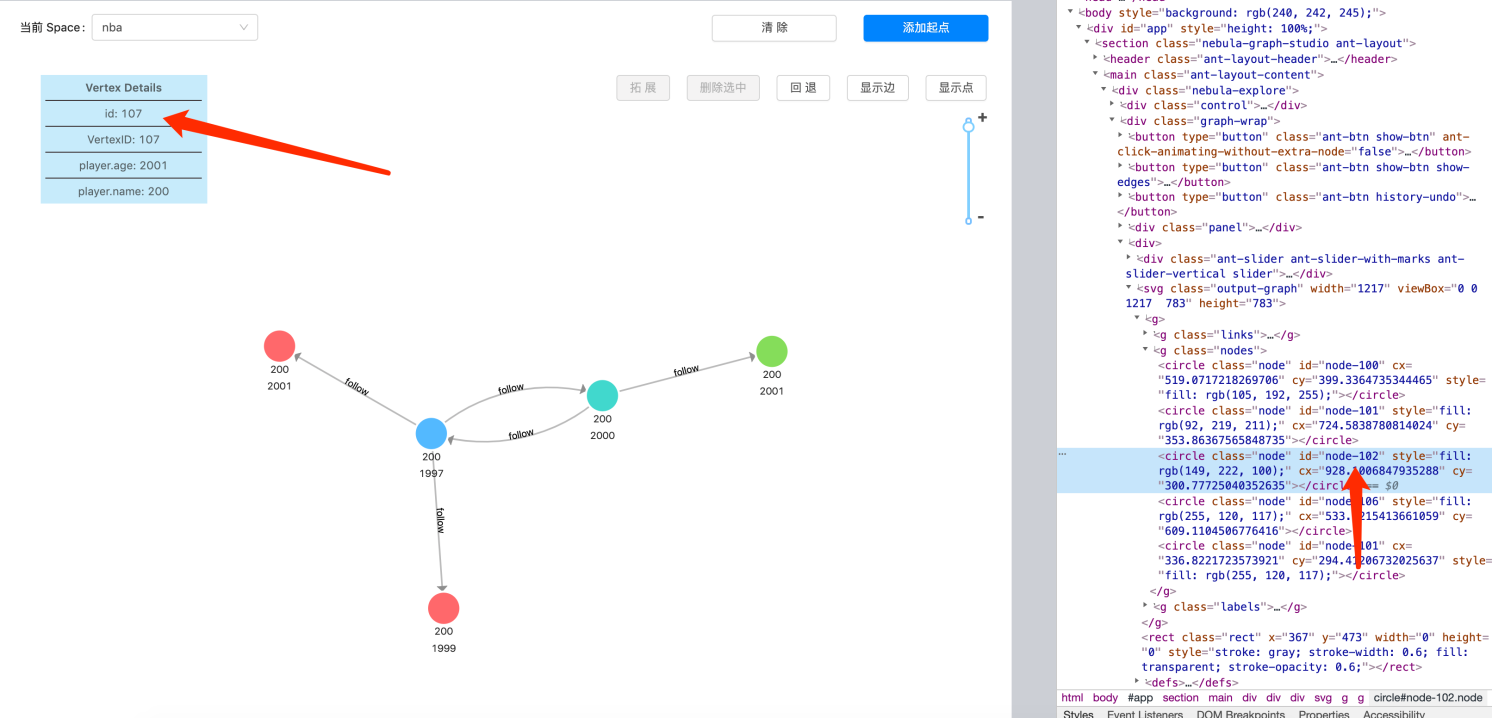# D3.js 力导向图的显示优化（二）- 自定义功能## 构建 D3.js 力导向图

``````this.force = d3
.forceSimulation()
// 为节点分配坐标
.nodes(data.vertexes)
// 连接线
// 整个实例中心
.force('center', d3.forceCenter(width / 2, height / 2))
// 引力
.force('charge', d3.forceManyBody().strength(-20))
// 碰撞力 防止节点重叠
``````## 支持删除任意选中功能

• 数据元素个数多于 `DOM` 元素个数
• 数据元素与 `DOM` 元素个数一样
• 数据元素个数少于 `DOM` 元素个数``````  componentDidUpdate(prevProps) {
const { nodes } = this.props;
if (nodes.length < prevProps.nodes.length) {
const removeNodes = _.differenceBy(
prevProps.nodes,
nodes,
(v: any) => v.name,
);
removeNodes.forEach(removeNode => {
d3.select('#name_' + removeNode.name).remove();
});
} else {
this.labelRender(this.props.nodes);
}
}
``````

## 支持按钮缩放功能

`````` <svg
width={width}
viewBox={`0 0 \${width * (1 + scale)}  \${height * (1 + scale)}`}
height={height}
>
{/*****/}
</svg>
``````

`````` const { width, height } = this.props;
const scale = (100 - zoomSize) / 100;
const offsetX = width * (scale / 2);
const offsetY = height * (scale / 2);
// 操作节点边父元素 DOM <g/> 的偏移
d3.select(this.circleRef).attr(
'transform',
`translate(\${offsetX} \${offsetY})`,
);
``````